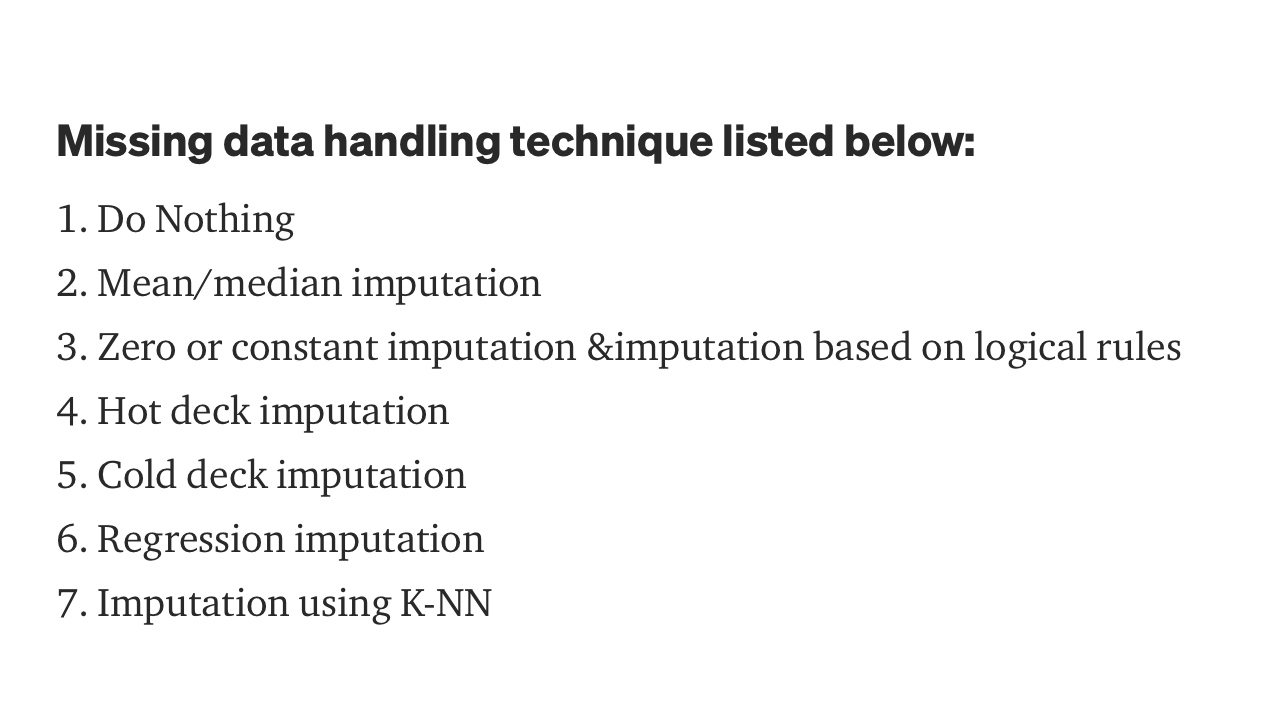# What are all technique to handle the missing data in a dataset??

First of all, we will try to understand the type of missing data, mainly there are 3 types of missing data:

1. Missing completely at random(MCAR)- When any missing values have no hidden dependency on any other variable or any characteristic of observations.
2. Missing at random(MAR) — Probability of missing value depends on the characteristics of observable data.
3. Missing not at random(MNAR or NMAR(Not missing at random)) — When the missing values depend on both characteristics of the data and also on missing values.

Missing data handling technique listed below:

1. Do Nothing
2. Mean/median imputation
3. Zero or constant imputation &imputation based on logical rules
4. Hot deck imputation
5. Cold deck imputation
6. Regression imputation
7. Imputation using K-NN

DoNothing-Some algorithms can factor in the missing values and learn the best imputation values for the missing data based on the training loss reduction so let the algorithm handle the missing data for example XGBoost. Some algorithms have the option to just ignore for example LightGBM — use_missing=false). Some algorithms will panic and throw an error complaining about the missing values (ie. Scikit learn — LinearRegression). In this case, you will need to handle the missing data and clean it before feeding it to the algorithm.

Mean/median/mode imputation Calculate the mean/median of the non-missing column values in a column and then replace the missing values within each column separately and independently from the others. mean/median can only be used with numeric data,mode can works with categorical data too.

Most frequent and zero or constant imputation or imputation based on logical rules — Most Frequent is another statistical strategy to impute missing values.It works with categorical features (strings or numerical representations) by replacing missing data with the most frequent values within each column.

Zero or Constant imputation — as the name suggests — it replaces the missing values with either zero or any constant value you specify,Suppose in a dataset we are having DOB and age as a 2 feature and age or DOB is missing so based on the condition we can calculate fill the missing data.

Hot Deck Imputation — A randomly chosen value from an individual in the sample who has similar values on other variables(column).find all the sample subjects who are similar on other variables, then randomly choose one of their values on the missing variable. You can use ffill that uses last observation carried forward (LOCF) Hot Code Imputation.

Cold deck imputation — A systematically chosen value from an individual who has similar values on other variables(column).This is similar to Hot Deck in most ways, but removes the random variation.

Regressing Imputation — The predicted value obtained by regressing the missing variable on other variables, instead of just taking the mean, you’re taking the predicted value, based on other variables. This preserves relationships among variables involved in the imputation model, but not variability around predicted values.Use Impute library in python for regression impution.

Imputation using K-NN — The k nearest neighbors is an algorithm that is used for simple classification, this algorithm uses ‘feature similarity’ to predict the values of any new data points, meaning that the new point is assigned a value based on how closely it resembles the points in the training set.We will perform similar kind of prediction to conform the missing datapoint using Impyute library in python, which provides a simple and easy way to use KNN for imputation,It creates a basic mean impute then uses the resulting complete list to construct a KDTree. Then, it uses the resulting KDTree to compute nearest neighbors (NN). After it finds the K-NN, it takes the weighted average of them.

1. See that 👏 icon? Send my article some claps
2. Connect with me via linkedin,github and on medium👈
3. source code you can find it here 👈Gautam_kmr

Hi I am data scientist and blogger from India…all technique to handle the missing data

Missing completely at random(MCAR)- When any missing values have no hidden dependency on any other variable or any characteristic of observations. Missing at random(MAR) — Probability of missing value depends on the characteristics of observable data. Missing not at random(MNAR or NMAR(Not missing at random)) — When the missing valuesSend a \$0.01 microtip in crypto to the author, and earn yourself as you read!

20% to author / 80% to me.
We pay the tips from our rewards pool.